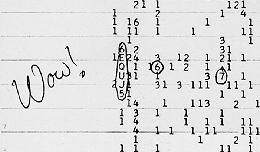Explanation of the Code "6EQUJ5" On the Wow! Computer Printout By Jerry EhmanThe photo of the computer printout of the Wow! source shows not only my handwritten comment ("Wow!") but also the circling of the 6 characters "6EQUJ5" lined up vertically in a column. What is the meaning of this code? Each of the first 50 columns of the computer printout shows the successive values of intensity (or power) received from the Big Ear radio telescope in each channel (10 kHz wide) in successive 12-second intervals (10 seconds was used for actual sampling and another approximately 2 seconds was needed for computer processing). In order to conserve space on the printout, Bob Dixon and I decided to use a coding method that would result in only one alphanumeric (i.e., either alphabetic or numeric) character for each intensity. The computer was programmed to keep a continuously-updated account for each channel of a baseline value and an rms value (rms stands for "root mean square", which is equivalent to the statistical term "standard deviation"). The actual intensity (after the baseline value was subtracted out) was then divided by the rms value to obtain a scaled value (i.e., the number of standard deviations above the baseline). Since there was space for only one character to be displayed, we decided to take only the integer value of this scaled intensity for values in the range 0 to 9.999... . The truncated value of zero was printed as a blank (space). The truncated value of 1, 2, 3, 4, 5, 6, 7, 8, and 9 were printed directly. For scaled intensities of 10 to 35, inclusive, the capital letters of the alphabet were used. Thus a truncated value of 10 was printed as an "A", 11 as a "B", etc. If the scaled intensity ever got to 36.0 or above, the program would simply start over again at zero (e.g., a truncated value of 38 would be printed the same as that of 38-35=3, namely a "3"). Thus, the "6EQUJ5" code in channel 2 means successive intensities as follows: 6 --> the range 6.0 - 6.999... E --> the range 14.0 - 14.999... Q --> the range 26.0 - 26.999... U --> the range 30.0 - 30.999... J --> the range 19.0 - 19.999... 5 --> the range 5.0 - 5.999... The value "U", meaning the range 30.0 - 30.999..., was the largest value ever seen. We do not believe that the intensity ever got above 31.0 and hence no rollover (subtraction of 35) ever occurred. It would have been easy to spot in a sequence of 6 or 7 numbers that should follow the antenna pattern of the telescope. The six successive values in channel 2 fit the antenna pattern of Big Ear very well. I have also done a correlation analysis of the six data points with the mathematical functions: (1) gaussian = normal curve; and (2)(sin(x)/x)^2. The data fit each of those two functions very well with correlation coefficients of over 0.99 (i.e., almost a perfect fit). I also fit the data to each of the two actual antenna patterns (of the two horns) using the moderately strong radio source OY372. The correlation coefficients were again over 0.99. There was not enough difference between the two correlation coefficients to determine which horn the Wow! source was received in.Copyright © 1996-2008 Ohio State University Radio Observatory and North American AstroPhysical Observatory. Originally designed by Point & Click Software, Inc. Last modified: February 20, 2008.